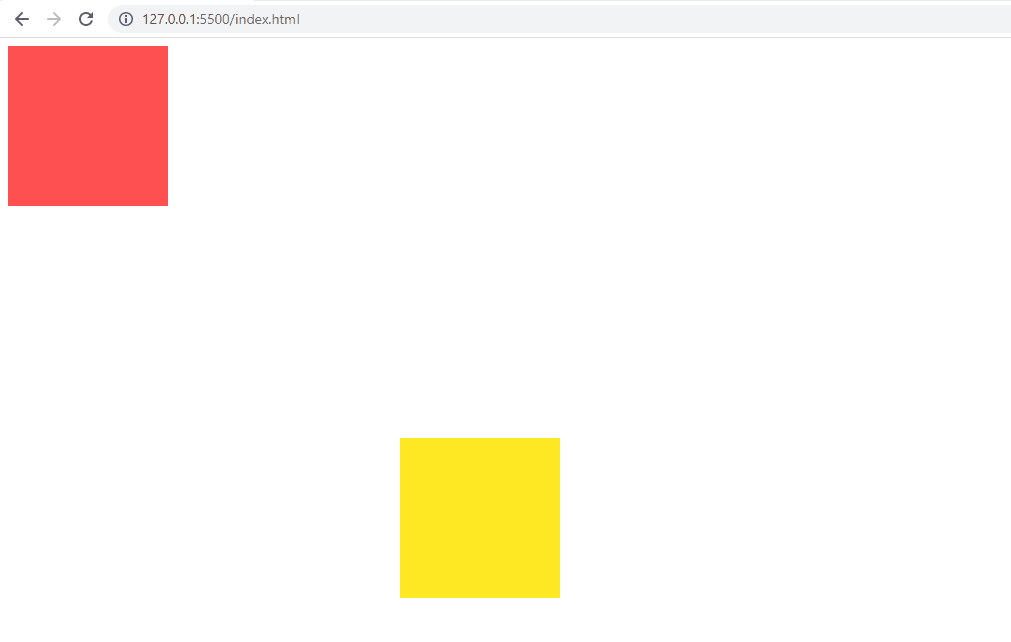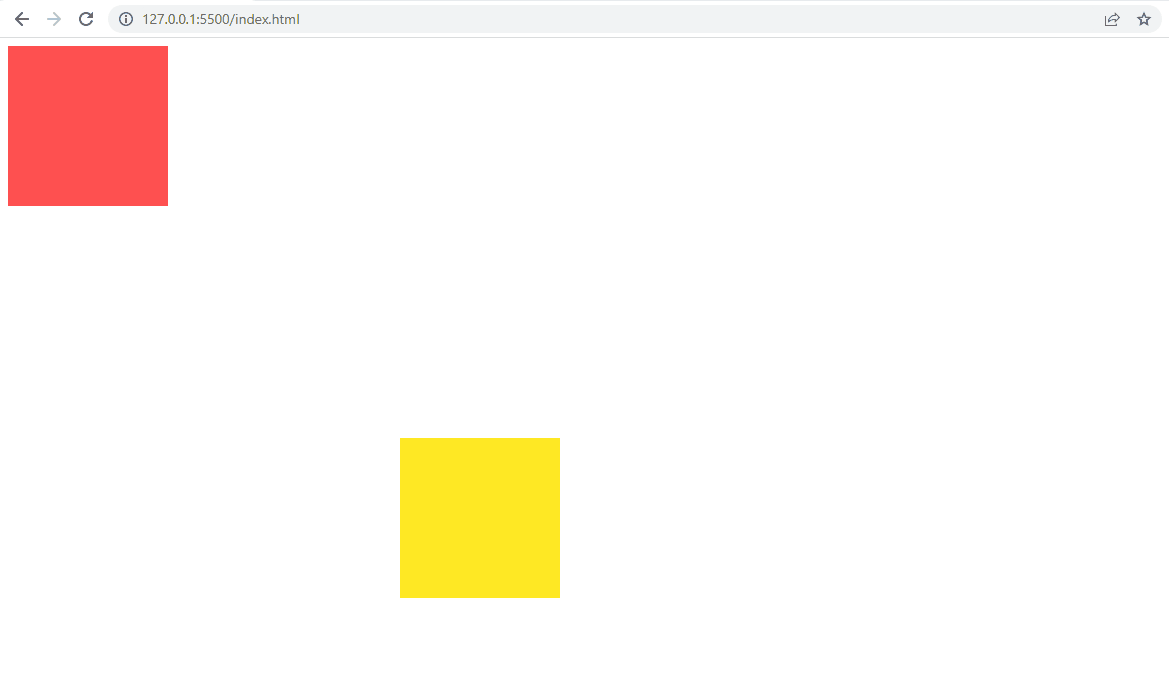# 在 JavaScript 中实现冲突检测

## 在 JavaScript 中实现冲突检测

``````<body>
<div class="square_1"></div>
<div class="square_2"></div>
</body>
``````

``````.square_2{
width: 160px;
height: 160px;
position: absolute;
left: 400px;
top: 400px;
background-color:rgb(255, 233, 36);
}

.square_1{
position: absolute;
width: 160px;
height: 160px;
background-color:rgb(255, 80, 80);
font-size: 1.5em;
z-index: 1;
}
````````````var square_1 = document.getElementsByClassName('square_1');
var square_2 = document.getElementsByClassName('square_2');

var square_1_width = Number((getComputedStyle(square_1).width).split("px"))/2;
var square_2_width = Number((getComputedStyle(square_2).width).split("px"))/2;

var square_1_height = Number((getComputedStyle(square_1).height).split("px"))/2;
var square_2_height = Number((getComputedStyle(square_2).height).split("px"))/2;
``````

``````square_1.addEventListener('mousemove', (e)=>{
square_1.style.left = (e.clientX - square_1_width) + "px";
square_1.style.top = (e.clientY - square_1_height) + "px";

checkCollision();
});
```````e.clientX` 和 `e.clientY` 给出了鼠标移动的右上角和右下角位置。

``````function checkCollision(){
let x_pos_sq_1 = Number((getComputedStyle(square_1).left).split("px")) ;
let y_pos_sq_1 = Number((getComputedStyle(square_1).top).split("px"));

let x_pos_sq_2 = Number((getComputedStyle(square_2).left).split("px")) ;
let y_pos_sq_2 = Number((getComputedStyle(square_2).top).split("px"));

let leftPos = x_pos_sq_1 + square_1_width > x_pos_sq_2 - square_2_width;
let rightPos = x_pos_sq_1 - square_1_width < x_pos_sq_2 + square_2_width;

let topPos = y_pos_sq_1 + square_1_height > y_pos_sq_2 - square_2_height;
let bottomPos = y_pos_sq_1 - square_1_height < y_pos_sq_2 + square_2_height;

if(leftPos && rightPos && topPos && bottomPos){
square_1.innerHTML = "Collision occured";
square_2.style.border = "5px solid rgb(24, 251, 240)";
}
else{
square_1.innerHTML = "";
square_2.style.border = "none";
}
}
``````## 在 JavaScript 中实现冲突检测

``````<body>
<div class="square_1"></div>
<div class="square_2"></div>
</body>
``````

``````.square_2{
width: 160px;
height: 160px;
position: absolute;
left: 400px;
top: 400px;
background-color:rgb(255, 233, 36);
}

.square_1{
position: absolute;
width: 160px;
height: 160px;
background-color:rgb(255, 80, 80);
font-size: 1.5em;
z-index: 1;
}
````````````var square_1 = document.getElementsByClassName('square_1');
var square_2 = document.getElementsByClassName('square_2');

var square_1_width = Number((getComputedStyle(square_1).width).split("px"))/2;
var square_2_width = Number((getComputedStyle(square_2).width).split("px"))/2;

var square_1_height = Number((getComputedStyle(square_1).height).split("px"))/2;
var square_2_height = Number((getComputedStyle(square_2).height).split("px"))/2;
``````

``````square_1.addEventListener('mousemove', (e)=>{
square_1.style.left = (e.clientX - square_1_width) + "px";
square_1.style.top = (e.clientY - square_1_height) + "px";

checkCollision();
});
```````e.clientX` 和 `e.clientY` 给出了鼠标移动的右上角和右下角位置。

``````function checkCollision(){
let x_pos_sq_1 = Number((getComputedStyle(square_1).left).split("px")) ;
let y_pos_sq_1 = Number((getComputedStyle(square_1).top).split("px"));

let x_pos_sq_2 = Number((getComputedStyle(square_2).left).split("px")) ;
let y_pos_sq_2 = Number((getComputedStyle(square_2).top).split("px"));

let leftPos = x_pos_sq_1 + square_1_width > x_pos_sq_2 - square_2_width;
let rightPos = x_pos_sq_1 - square_1_width < x_pos_sq_2 + square_2_width;

let topPos = y_pos_sq_1 + square_1_height > y_pos_sq_2 - square_2_height;
let bottomPos = y_pos_sq_1 - square_1_height < y_pos_sq_2 + square_2_height;

if(leftPos && rightPos && topPos && bottomPos){
square_1.innerHTML = "Collision occured";
square_2.style.border = "5px solid rgb(24, 251, 240)";
}
else{
square_1.innerHTML = "";
square_2.style.border = "none";
}
}
``````## 在 JavaScript 中实现冲突检测

``````<body>
<div class="square_1"></div>
<div class="square_2"></div>
</body>
``````

``````.square_2{
width: 160px;
height: 160px;
position: absolute;
left: 400px;
top: 400px;
background-color:rgb(255, 233, 36);
}

.square_1{
position: absolute;
width: 160px;
height: 160px;
background-color:rgb(255, 80, 80);
font-size: 1.5em;
z-index: 1;
}
````````````var square_1 = document.getElementsByClassName('square_1');
var square_2 = document.getElementsByClassName('square_2');

var square_1_width = Number((getComputedStyle(square_1).width).split("px"))/2;
var square_2_width = Number((getComputedStyle(square_2).width).split("px"))/2;

var square_1_height = Number((getComputedStyle(square_1).height).split("px"))/2;
var square_2_height = Number((getComputedStyle(square_2).height).split("px"))/2;
``````

``````square_1.addEventListener('mousemove', (e)=>{
square_1.style.left = (e.clientX - square_1_width) + "px";
square_1.style.top = (e.clientY - square_1_height) + "px";

checkCollision();
});
```````e.clientX` 和 `e.clientY` 给出了鼠标移动的右上角和右下角位置。

``````function checkCollision(){
let x_pos_sq_1 = Number((getComputedStyle(square_1).left).split("px")) ;
let y_pos_sq_1 = Number((getComputedStyle(square_1).top).split("px"));

let x_pos_sq_2 = Number((getComputedStyle(square_2).left).split("px")) ;
let y_pos_sq_2 = Number((getComputedStyle(square_2).top).split("px"));

let leftPos = x_pos_sq_1 + square_1_width > x_pos_sq_2 - square_2_width;
let rightPos = x_pos_sq_1 - square_1_width < x_pos_sq_2 + square_2_width;

let topPos = y_pos_sq_1 + square_1_height > y_pos_sq_2 - square_2_height;
let bottomPos = y_pos_sq_1 - square_1_height < y_pos_sq_2 + square_2_height;

if(leftPos && rightPos && topPos && bottomPos){
square_1.innerHTML = "Collision occured";
square_2.style.border = "5px solid rgb(24, 251, 240)";
}
else{
square_1.innerHTML = "";
square_2.style.border = "none";
}
}
``````